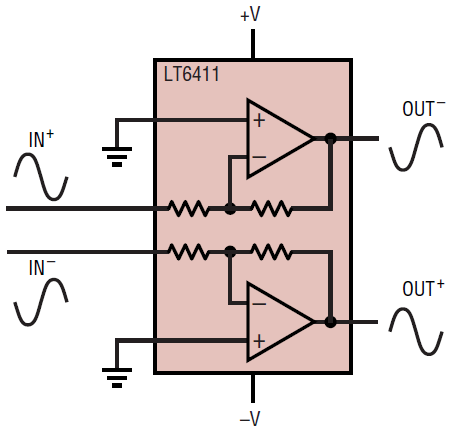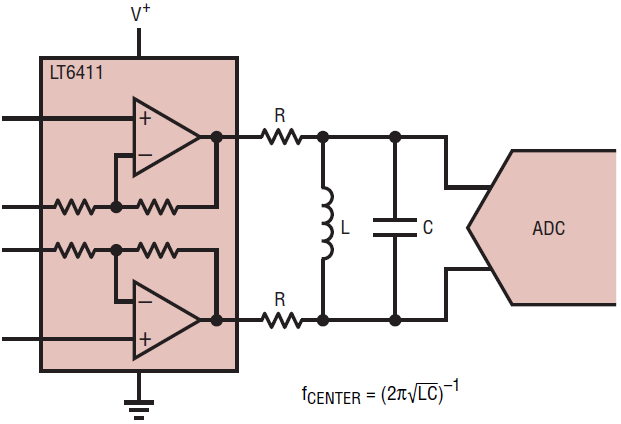# 650MHz Selectable-Gain Amplifier/Differential ADC Driver Has Small Form but Many Functions

Introduction

Operational amplifiers have always been an important part of an analog designer’s bag of tools. Even the most basic tools can be improved, and some recent advancements have increased the utility of the workhorse op amp. For instance, the advent of dual and quad op amp packages allowed engineers to produce a variety of applications from a single device. The new LT6411 selectable-gain differential amplifier/ADC driver makes a good thing better by adding internal gain and feedback resistors and an easy-to-use flow-through pin layout.

The LT6411 can produce gains of 1, –1, and 2 with no external components. The dual amplifiers inside the LT6411 allow for easy single-ended-to-differential conversion for driving high-speed analog-to-digital converters (ADC). The wide bandwidth (650MHz), low distortion (–77dBc harmonic distortion at 30MHz) and high slew rate (3300V/µs) preserve signal fidelity even at high frequencies, while the low supply current (16mA total) enables the LT6411 to be used in power-critical high-speed signal chain applications. Form factor is also not an issue—all of these features fit into the tiny 3mm × 3mm 16-pin QFN package. When blazing fast speed, low power, or the flexibility of selectable gains is necessary, a circuit designer need only reach into his bag of tools and pull out the LT6411.

Internal Topology and Gain Selection

The LT6411 contains two internal current-feedback amplifiers with matched feedback and gain resistors. The integrated 370Ω resistors take the guesswork out of selecting the optimal feedback resistor for good AC response. Another common source of frustration with current-feedback amplifiers is maintaining a tight PC board layout to prevent excessive capacitance at the inverting input node. This node is internal to the LT6411, so the layout of the circuit board is nearly optimal from the get-go.

Selecting between the basic gain configurations of the LT6411 is a simple matter of pin strapping. Figure 1 shows the standard non-inverting gain of 2 configuration, with differential input and output. Figure 2 shows the non-inverting gain of 1 configuration, and Figure 3 shows an inverting gain of 1 configuration. Note that in Figure 2, the non-inverting inputs are tied together with the gain resistor. In theory, the gain resistor pin could be left floating, but in practice, the parasitic pin and pad capacitances cause the gain to peak up to 6dB at high frequencies, which causes excessive ringing in the transient response. At 650MHz (the bandwidth of the LT6411), 2pF of parasitic capacitance has a reactance of just 122Ω.Figure 1. Standard non-inverting gain of 2 configuration, shown with split supplies.Figure 2. Standard non-inverting gain of 1 configuration, shown with dual supplies. The gain resistors, shown here tied to the inputs, should not be left floating (see text).Figure 3. Standard inverting gain of 1 configuration, shown with dual supplies.

Single-Supply Operation and Level-Shifting

The LT6411 operates on a wide supply voltage range, from 4.5V–12.6V. Figures 1–3 show the part with dual supplies. However, the LT6411 performance remains excellent with a single supply, especially important for many common applications such as high speed ADC driving, where only a single supply is available. If the input signals into the LT6411 are already DC level-shifted above ground so that the input and output common-mode ranges are met (the input operates to within 1V of the supplies, the output with a 1k load swings to within 1.3V of the supplies), no additional work needs to be done. However, if the input signal is AC-coupled or centered around ground, then level-shifting is necessary. This section presents some of the methods to level-shift the input and output voltages of the LT6411.

Figure 4 shows a center-tapped transformer providing the DC voltages necessary for single-supply operation. The input signal (shown single-ended, but can also be differential by driving the other end of the primary) is provided through the transformer primary, and the transformer secondary presents a balanced signal to the LT6411. If the input signal is coming from a 50Ω signal source, the two 24.9Ω resistors provide the appropriate termination. Note that the DC voltage provided at the center tap of the transformer is VO(DC)/2, due to the fact that the LT6411 has a non-inverting gain of 2. Alternatively, the two gain resistor pins could be AC-coupled to ground through a capacitor, and the DC gain of the LT6411 would be unity. The total differential gain in this configuration is 2; if a differential gain of 1 is desired, simply tie the gain resistor pins to the corresponding non-inverting input pins.Figure 4. DC level-shifting through the center tap of a transformer for single-supply operation. The DC voltage source at the center tap should serve as a low-impedance AC ground. The two 24.9Ω resistors provide a 50Ω termination, if necessary.

For a single-ended input, Figure 5 shows a simple AC-coupled method of providing the correct input and output DC levels for the LT6411. The input is AC-coupled through a large capacitor (typically 0.1µF or larger), and the total differential gain at the output is +2. The DC voltage is provided through the second non-inverting input.Figure 5. A simple AC-coupled method of providing DC level shifting. The DC voltage source, which does not need to have a low impedance, is provided at the non-inverting input of the second amplifier. The AC input impedance of this circuit is 370Ω.

In the case of a differential inputs, Figure 6 shows a similar configuration, where the two inputs are AC-coupled to the LT6411, and the DC level is provided through two resistors. The choice of size for the resistance R is a trade-off between loading the differential inputs (for smaller values of R) and increasing voltage offset and noise (for larger values of R, due to the input bias currents and current noise in the non-inverting inputs). Values of R up to 10k work well in practice. The DC and AC inputs both have a gain of 2 in this configuration. For a gain of 1, simply tie the gain resistor pins to the corresponding non-inverting input pins.Figure 6. A DC voltage source and two resistors sets the DC input and output level of the LT6411. The two resistors must be large enough not to overload the inputs.

Figures 4–6 all have a lower frequency limitation, defined by either the transformer’s magnetizing inductance or the size of the AC-coupling capacitor. What if voltage level-shifting and response down to zero Hertz (DC) is necessary? Figure 7 shows one method of level-shifting and maintaining signal response down to DC. The RA-RB resistive dividers set the input common-mode voltage for the LT6411. Choose RA and RB so that the common-mode voltage at the input of the LT6411 is the same as VO(DC), the desired output common-mode voltage. The gain of the LT6411 with the resistive divider is 2 • RB/(RA + RB), and this circuit has a response to DC. If the input DC common-mode level (VI, DC) is greater than the VO,DC, then RB should be attached to ground instead of the positive supply.Figure 7. Two identical resistive dividers (RA and RB) shift the DC level of the input (and output) to within the limits of the LT6411. VO,DC must have a low impedance at the frequencies of interest, and must be capable of sourcing and sinking currents through the internal resistors.

Modern high resolution, high speed ADCs typically feature differential inputs with capacitive sample-and-hold circuits. Driving these inputs requires an amplifier with high bandwidth, fast settling times, good transient response and good distortion performance at the frequencies of interest. The figures in this section show various configurations designed to get the maximum performance out of the LT6411.

The LT6411 is optimized for driving 12-bit and 14-bit high-speed converters such as the 14-bit, 80Msps LTC2249. For many applications, the only additional recommended interfacing components would be small series resistors, to help isolate the LT6411 from the ADC’s capacitive input. Figure 8 shows the simplest configuration for the LT6411 driving the ADC. The single supply operation means that either the inputs must have an appropriate DC common-mode level (that is, VDC in the Figure 11 must be appropriate for both the amplifier and the ADC), or that one of the techniques from the previous section must be used to level-shift the input.

If dual supplies are used for the LT6411, then interfacing with a single-supply ADC might require AC-coupling at the output, as shown in Figure 9. The DC level of the ADC input is established by VDC and two 499Ω resistors.Figure 9. Level-shifting the LT6411 output to within the input common-mode range of the single-supply ADC. The resistors used must be large enough not to excessively load the outputs of the LT6411. Some ADCs have a VCM output that can be used for VDC.

Figure 8’s circuit, though simple, has an important drawback: all of the wideband noise of the amplifier couples into the ADC input, and thus degrades the signal-to-noise ratio (SNR) of the signal. In most cases, the input signal is limited to some frequency band less than the DC–650MHz bandwidth of the LT6411; thus, any extra bandwidth beyond that introduces unnecessary noise. The simplest approach to fixing this problem appears in Figure 10. A single shunt capacitor creates an RC lowpass filter that limits the noise bandwidth of the amplifier output, improving the SNR.Figure 10. An RC lowpass filter is shown at the output of the LT6411. Reducing the bandwidth at the output also reduces the amount of wideband noise seen by the ADC.

For an even sharper cutoff lowpass filter, Figure 11 shows a more involved approach. The inductors and capacitor create a second-order lowpass filter, with R1 ensuring that the frequency peaking is not excessive. R2’s primary function is to ensure that the ADC inputs do not see too high of a source impedance. Figure 12 shows some sample values, configured for a cutoff of around 50MHz and almost no gain peaking near the cutoff frequency. Simulating the filter is a good way to determine optimal component values, especially when taking into account the series resistance of the inductor and component tolerances.Figure 11. A second-order LC lowpass filter, offering a flatter passband and sharper stopband rolloff than an RC filter. The series resistor R1 controls peaking near the cutoff frequency, and the parallel resistor R2 ensures that the ADC sees a low source impedance at very high frequencies.Figure 12. The circuit of Figure 11, configured for a cutoff frequency of around 50MHz.

Some high frequency applications contain very narrow-band signals, where a bandpass filter would provide the best noise limiting, and thus the highest SNR. Figure 13 shows a simple RLC bandpass filter. The value of R determines the quality factor (Q) of the filter—the larger the resistor value, the more narrow-band the filter. This comes at the cost of more pass-band loss (depending on the parasitic components of the inductor and capacitor) and higher sensitivity to component tolerances and variations. As the bandpass filter gets narrower, a small shift in center frequency can significantly attenuate the desired output signal.Figure 13. For narrow-band applications, an LC bandpass filter does an excellent job limiting the noise at the ADC input while maintaining a low component count. The series resistor affects the width of the pass-band and the pass-band attenuation.

Conclusion

The LT6411 selectable-gain amplifier/ADC driver is extremely flexible, featuring a multitude of possible configurations with a minimal number of external components. The LT6411 is a dual op amp, a differential ADC driver, and a selectable-gain amplifier all in one. In most basic dual op amp functions, all that is required is a few power supply bypass capacitors to get excellent AC performance to over 600MHz. In addition, the LT6411 comes in the tiny 3mm × 3mm 16-lead QFN package and consumes only 16mA total. The LT6411 also has a shutdown feature that reduces the power supply current to 700µA total. With this unique set of features, the LT6411 can provide myriad functions without breaking the budget of size or power.